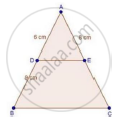Share

# In δAbc, D and E Are Points on the Sides Ab and Ac Respectively Such that De || Bc If Ad = 6 Cm, Db = 9 Cm and Ae = 8 Cm, Find Ac. - Mathematics

Course

#### Question

In ΔABC, D and E are points on the sides AB and AC respectively such that DE || BC

If AD = 6 cm, DB = 9 cm and AE = 8 cm, find AC.

#### SolutionWe have,

DE || BC

Therefore, by basic proportionally theorem,

We have

"AD"/"DB"="AE"/"EC"

rArr6/9=8/"EC"

rArr2/3=8/"EC"

rArr"EC"=(8xx3)/2

⇒ EC = 12 cm

⇒ Now, AC = AE + EC = 8 + 12 = 20 cm

∴ AC = 20 cm

Is there an error in this question or solution?

#### APPEARS IN

RD Sharma Solution for Class 10 Maths (2018 (Latest))
Chapter 7: Triangles
Ex. 7.2 | Q: 1.01 | Page no. 19
RD Sharma Solution for Class 10 Maths (2018 (Latest))
Chapter 7: Triangles
Ex. 7.2 | Q: 1.01 | Page no. 19

#### Video TutorialsVIEW ALL 

Solution In δAbc, D and E Are Points on the Sides Ab and Ac Respectively Such that De || Bc If Ad = 6 Cm, Db = 9 Cm and Ae = 8 Cm, Find Ac. Concept: Basic Proportionality Theorem Or Thales Theorem.
S